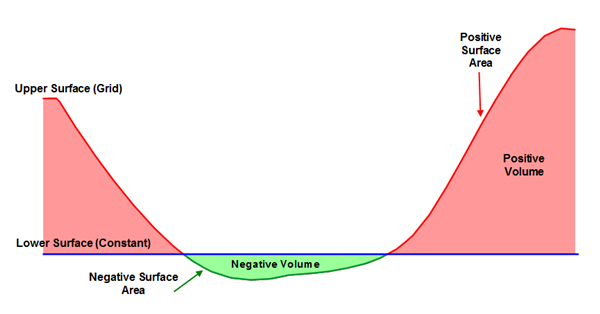# Surface Area Calculations

The surface area is the actual area of the surface. Positive Surface Area corresponds to the area of the surface where the upper surface is above the lower surface. Negative Surface Area corresponds to the area of the surface where the upper surface is below the lower surface.

## Example

As an example, consider a grid file representing the bottom of a pond, with the water level at a Z value of zero. If the grid representing the pond bottom is defined as the upper surface and Z = 0 is defined as the lower surface, you can determine the surface area of the bottom of the pond. Suppose you want to drain the pond and line it with plastic. You need to know the area of the plastic necessary to line the pond bottom. In this case, the negative surface area represents the surface of the pond bottom. This would tell us precisely the amount of plastic necessary to line the pond bottom.The green line displays the negative surface area of the pond. This value determines the amount of plastic needed to line the pond bottom in the example.